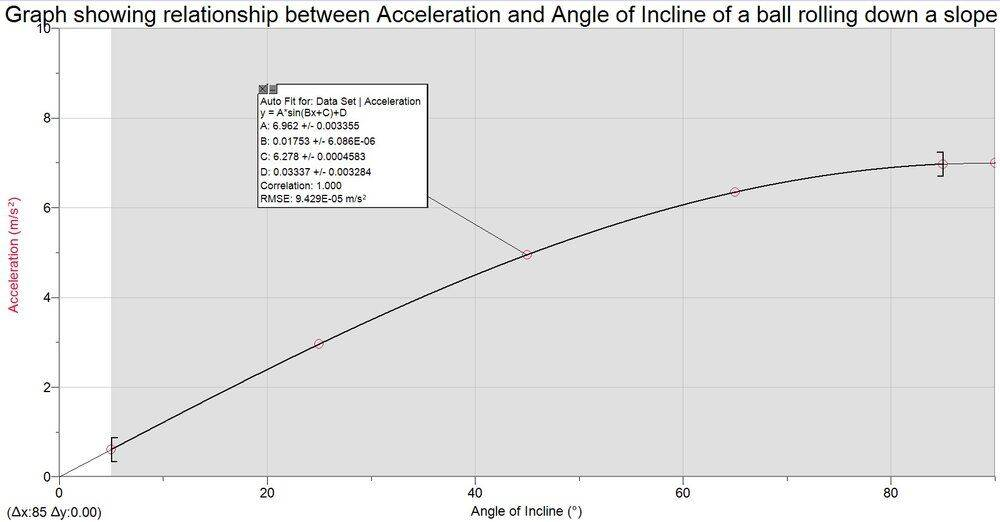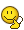# Why does acceleration increase by less as angle of slope increases?

Homework Statement:
why does acceleration of a solid sphere increase by less as the angle of incline increases?
Relevant Equations:
a = 5mgsin𝛳/7
This is true considering the rotational inertia of a solid sphere is (2/5)mr^2
My only guess is that this is due to air resistance. Below an example of the predicted graph:## Answers and Replies

etotheipi
Well, why don't you work out ##\frac{da}{d\theta}## and see how then consider how the gradient varies with ##\theta##?

Last edited by a moderator:
Well, why don't you work out ##\frac{da}{d\theta}## and see how then consider how the gradient varies with ##\theta##?

[Also, it's curious to see that your leading coefficient is ##5/7##, since for a solid sphere on a ramp it will be ##5/9##. We had a thread about this a short while ago, and determined that if the solid sphere instead rolled down a V-shaped track, the leading coefficient would be ##5/7##. I wondered... are you using a V-shaped track?]

That's interesting. I am not using a v-shaped track, just a flat ramp. I'm new to this platform, where can I find the thread?

etotheipi
Oh wait, hang on, I got it the wrong way around. The ##5/7## is correct for the flat ramp. Just ignore me. The thread's here if you want: https://www.physicsforums.com/threads/is-this-formula-for-a-ball-rolling-down-a-ramp-incorrect.994767/

Anyway, what about the ##da/d\theta## business?

I'm not sure I understand, if I am correct then that means a change in acceleration over the change in angle. Not sure where to go from there. Can't find an immediate relationship.

etotheipi
It's a derivative! Are you familiar with calculus, and do you know how to find the derivative of ##\sin{\theta}## with respect to ##\theta##?

It's a derivative! Are you familiar with calculus, and do you know how to find the derivative of ##\sin{\theta}## with respect to ##\theta##?

I'm afraid I have gone too far down a rabbit hole. I could work it out for each angle and plot it on a graph and find the slope but I'm not sure how to do so otherwise.

etotheipi
Okay. What you would have ended up with is$$a = \frac{5}{7}g\sin{\theta} \implies \frac{da}{d\theta} = \frac{5}{7} g \cos{\theta}$$Here ##\frac{da}{d\theta}## is the rate of change of ##a## w.r.t ##\theta##, i.e. the gradient of the graph you posted. Notice that as ##\theta## gets closer to ##90^o##, ##\cos{\theta}## get closer to zero, i.e. the gradient gets smaller and your curve gets flatter and flatter! This is the more precise way of saying that "equal increments in ##\theta## give diminishing returns in ##a## as ##\theta## gets larger".

Last edited by a moderator:
•Jojo-11
jbriggs444
Science Advisor
Homework Helper
The m should be dropped from the above, of course. It would appear in a calculation of net force, but not acceleration.

•etotheipi
Okay. What you would have ended up with is$$a = \frac{5}{7}mg\sin{\theta} \implies \frac{da}{d\theta} = \frac{5}{7} mg \cos{\theta}$$Here ##\frac{da}{d\theta}## is the rate of change of ##a## w.r.t ##\theta##, i.e. the gradient of the graph you posted. Notice that as ##\theta## gets closer to ##90^o##, ##\cos{\theta}## get closer to zero, i.e. the gradient gets smaller and your curve gets flatter and flatter! This is the more precise way of saying that "equal increments in ##\theta## give diminishing returns in ##a## as ##\theta## gets larger".

I can see that but is there an explanation behind why the ball accelerates by less at the same increments for greater angles like increased air resistance or friction or is this just a given and we don’t know?

etotheipi
I can see that but is there an explanation behind why the ball accelerates by less at the same increments for greater angles like increased air resistance or friction or is this just a given and we don’t know?

Not really. That expression for acceleration is derived by considering friction, but that only really changes the leading coefficient of the term and not the general form of ##\alpha g \cos{\theta}##.

And of course, there is no ##m## in that expression for acceleration, as @jbriggs444 rightly points out•jbriggs444
SammyS
Staff Emeritus
Science Advisor
Homework Helper
Gold Member
Consider the acceleration of a block of mass, ##m##, sliding down an inclined plane (angle θ w.r.t. the horizontal).

The graph of acceleration vs. angle of inclination looks very much like yours.

haruspex
Science Advisor
Homework Helper
Gold Member
2020 Award
Homework Statement:: why does acceleration of a solid sphere increase by less as the angle of incline increases?
a = 5mgsin𝛳/7
The answer is right there in your equation: sin(θ), not θ.
What does a graph of y=sin(x) look like?